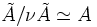# Gerstenhaber deformation

Let$A$ be an algebra (not necessarily associative) over a field$k$. Then, a Gerstenhaber deformation of$A$ is defined as an algebra$\tilde{A}$ over the ring$K[[\nu]]$ (ring of formal power series in the formal variable$\nu$), such that$\tilde{A}/\nu\tilde{A} \simeq A$. Two Gerstenhaber deformations are termed equivalent if they are isomorphic over$K[[\nu]]$ and a Gerstenhaber deformation is trivial if it is obtained by extending the base ring from$K$ to$K[[\nu]]$.